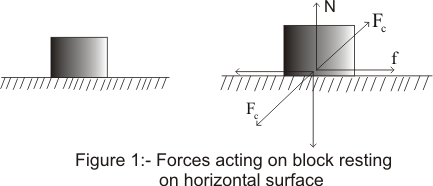# Force of Friction

## (1) force of friction

• We all know that what would happen if we slide a book kept along a horizontal table .Yes it would first sliding and then finally would come to rest
• This force opposing continuous motion of book on the table is called force of friction
• Whenever surface of one object slides over another ,both the bodies exerts force of friction on each other
• Here in this chapter,we will consider frictional forces acting between a pair of surfaces
• Frictional force comes into action whenever two surfaces of two bodies comes in contact with each other(Both the bodies could be moving or rest).Both the bodies exert a force on each other which is basically Electromagnetic in nature and this force is a contact force
• Magnitude of contact forces on two bodies are equal but they are opposite in direction
• Contact forces also depend on the nature of the surfaces of the two bodies kept in contact,how both the bodies are moving and all the forces acting on them

• Consider a block resting on the horizontal surface as shown below in the figure ,Now two bodies exert equal and opposite contact force on each other . Let Fc is the contact force both the bodies exert on each other• Direction of the contact force acting on a body need not necessarily be perpendicular to the surface of contact
• Contact force Fc can be resolved into two components.The component of Fc along the normal to the surface is called Normal
contact force and component parallel to the surface is called friction (f) as shown in fig (b)
• Friction is subdivided into two categories namely
1. Static Friction
2. Kinetic Friction
Static friction is experienced between the non moving surfaces i.e the bodies at rest and kinetic friction or dynamic friction is experienced between the moving surfaces or between the bodies in motion
• We shall now discuss both the static and kinetic friction in details